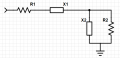# Derivation of impedance matching Q

#### sjgallagher2

Joined Feb 6, 2013
131
Hey all,
I need help finding an error in a derivation I'm doing. The equation
is used in impedance matching with L sections. Since I'm not one to blindly take an equation, like many students, I took to finding out where it comes from. I know that you're matching impedances, and in a circuit with two real loads and an L section, like this:you have two RC pairs. A resistance and reactance pair will always have a Q value. That means we find:
Qsource = X1/R1
Okay, all is going well. This is all supported in the book (RF Circuit Design, Bowick).We set Qsource=Qload. But we can't solve for a definite solution unless we have one more equation. Thus comes in the given equation:
Q = √(R2/R1 - 1)
So I'm thinking "Ok that looks unlike other stuff where did it come from". I assume the loads are matched such that:
R1+X1 = R2 || X2
and use the Q equations to replace the X1 and X2.
But that's where I stop and realize the Q equation for X1 and X2 assume that they are magnitudes only, when really they're complex values. The parallel combination of X2 and R2 will really come out to be complex as well, so replacing X2 with a real value might affect the answer.
I trudge on, with no idea of what effect this will have. I realized that the topic of Q being imaginary or real never came up. It must use the magnitudes instead.

So I did lots of algebra (due to mistakes, and trying again). I'm using Wolfram Alpha to check my work. But instead of
Q = √(R2/R1 - 1)
I get
Q = √(R2/R1) - 1
where the - 1 is outside of the square root. A far cry from the given equation. So I believe that my assumption in the parallel combination of a resistance and a reactance and then substituting out was faulty. In a way, I took [R2 || something] as a function f(X2) and replaced X2. But obviously that's wrong, if only by a small factor.

How can I fix this up? Where does the equation come from?

Note: I also have qualms about adding a resistance and a reactance, since that does not work mathematically. But again, I let it go. These are likely places for mistakes, but I don't know how to handle them, with the Q being real, and all that. I guess I'm all out of brain energy. SOS!

#### Tesla23

Joined May 10, 2009
537
I just did a quick derivation and I agree with Bowick.

You do know that you can't do a conjugate match with the two reactances being capacitive, they have to be opposite signs, so for your schematic one would have to be an inductor.

#### sjgallagher2

Joined Feb 6, 2013
131
Awesome to have someone help me out! I was worried the post would be lost. I have now figured out how to derive the equation. In case anybody else ever happens to need it I will explain it briefly. Using the image above I created the equation:
Rs + X = RL || X
but this is not quite correct, because it leaves out j from the equations. The real way to put it is:
Rs - jX = RL || jX
which becomes
Rs - jX = (RL * ||X||<90) / RL + jX
You have two equations for Q depending on series or parallel. The book describes it as Q = X/R and Q = R/X for series and parallel respectively. But this is not quite true. The real definition (for an inductor) is
Q = ωL/R
Now, X ≠ ωL, because you're leaving off the imaginary component. In fact, X = jωL. So to say that Q = X/R is not correct, but rather you should say Q = ||X|| / R.

Now we have a pair of equations for ||X|| (not X), which are:
||X|| = RsQ
||X|| = RL/Q
and we can use these by plugging them into the equation we got before as long as we can put it all in terms of ||X||. If you choose to leave out the phase contributions, which you should, you'll find that:
√(Rs^2 + ||X||^2) = (RL* ||X||) / √(RL^2 + ||X||^2).
Now you have everything in terms of some magnitude of X. Note I left out the negative signs only because they are cancelled out by the squares. In effect, it doesn't matter that they are opposite reactances in these calculations.
Filling in, you get
√(Rs^2 + (Rs^2)(Q^2) ) = (RL^2 ) / Q*√(RL^2 + (RL^2)/(Q^2) )
I simplified a small bit there but the point remains. Now work the algebra, it is not too bad. You pull an Rs and an RL from the √ 's, insert the Q into the square root on the right hand side, move the square root to the left hand side (they should be the same now) and you get
Q^2 + 1 = RL/Rs
which is the same as, look!
Q = √(RL/Rs - 1)
There's the proper equation. All is safe and sound in the world. Not that there was any doubt, but Bowick remains validated. You can now use this equation to match (real) impedances with complex L-sections. And good algebra practice too!

Last edited:

#### Tesla23

Joined May 10, 2009
537
You can simplify it a bit by just noting that this impedance transformation is generated by changing the parallel RC to a series equivalent and resonating out the effective series C.

For a parallel RC:

$$Z = \frac{R_P}{1+j\omega R_P C_P} = \frac{R_P}{1+jQ} = \frac{R_P(1-jQ)}{1+Q^2}$$

where $$Q = \omega R_P C_P$$

so the equivalent series R is
$$R_s = \frac{R_P}{1+Q^2}$$

and so
$$Q = \sqrt{\frac{R_P}{R_S} - 1}$$